HOME2020-03-04T20:12:08-05:00

## Welcome.

The TGDA group is an interdepartmental group at OSU, with faculty from with CSE, Mathematics, and Statistics whose interests span topics in the intersection of Topology, Geometry, Probability, Statistics, and Data Analysis.

## 2018 TRIPODS Workshop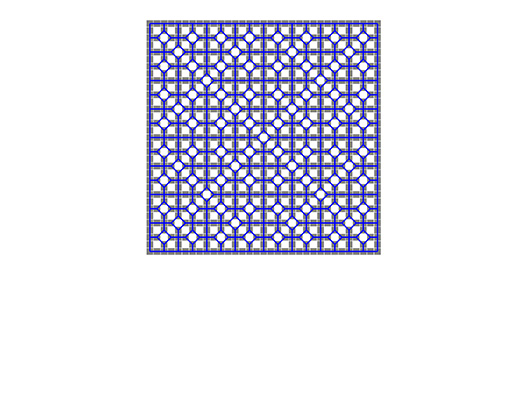Kahle and Roldán studied a question in extremal topological combinatorics: what is the maximal number of holes that a polyomino with n tiles can enclose? They proved that a certain infinite sequence of polyominoes is optimal. Curiously, the planar dual of the sequence converges to an aperiodic tiling of the plane. Image by Érika Roldán.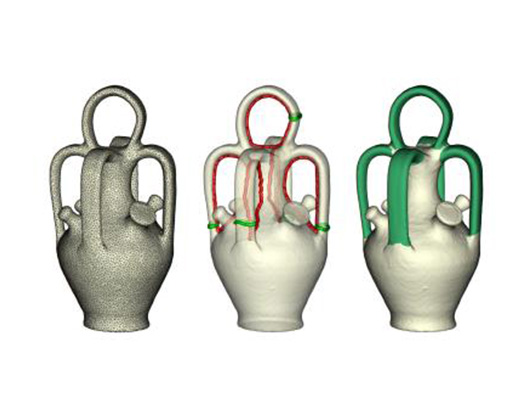Handle and Tunnel loops on surfaces computed by the algorithm of Dey, Li, Sun, Cohen-Steiner.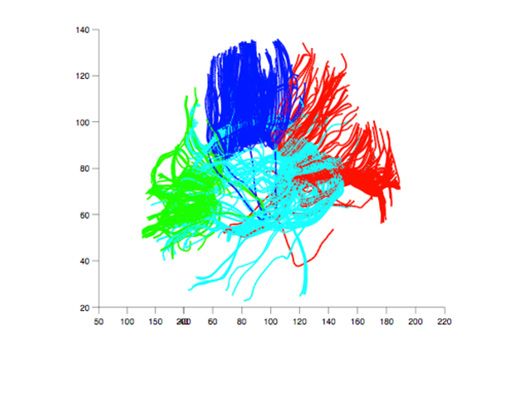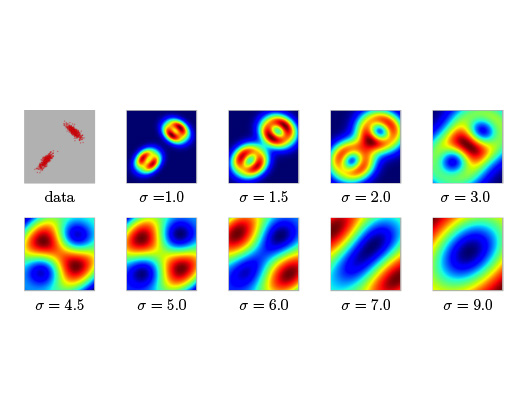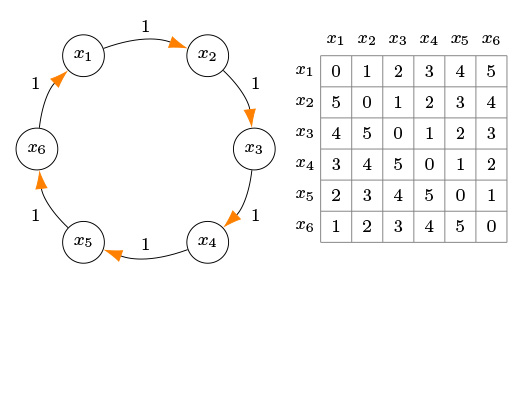Chowdhury and Memoli studied the extension of notions of persistent homology to the setting of directed networks. Image by S. Chowdhury.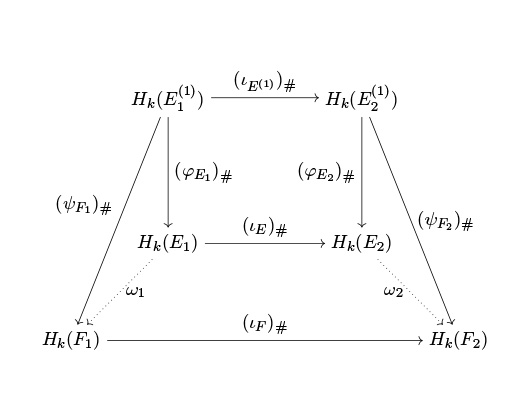A commutative diagram appearing in the proof of duality of Dowker persistent homology. Image by Samir Chowdhury and Facundo Memoli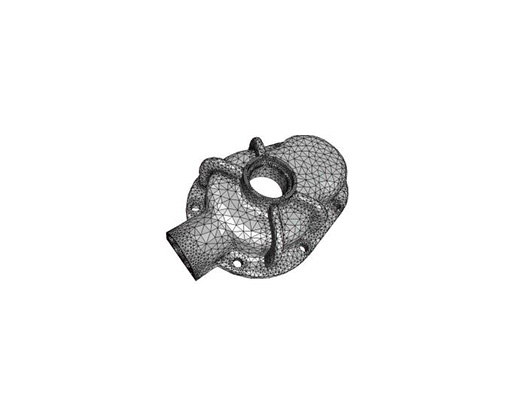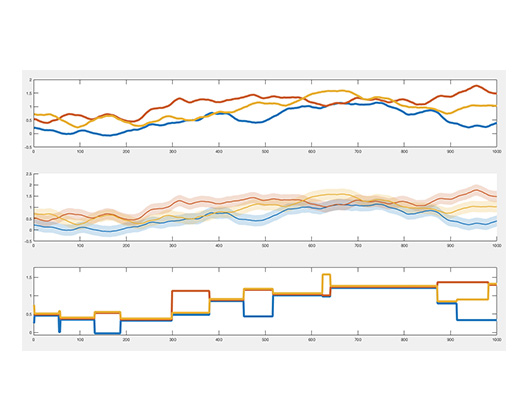A formigram: a data structure used for analysis of dynamic data. Image by Woojin Kim and Facundo Memoli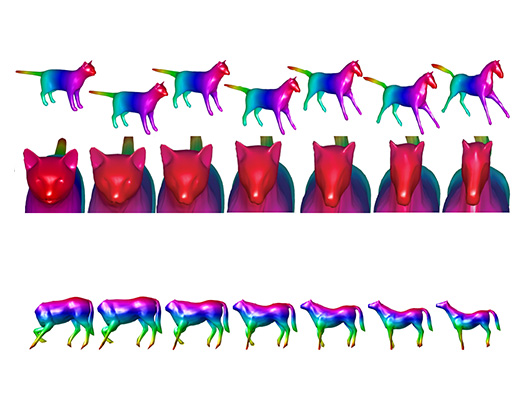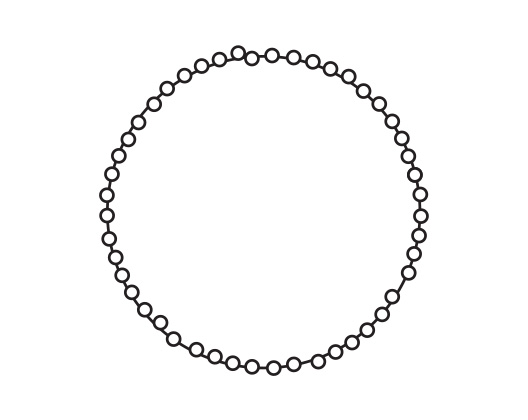A metric graph with small Gromov-Hausdorff to S^1 but very high Betti_1 number. Image by Facundo Memoli and Osman Berat Okutan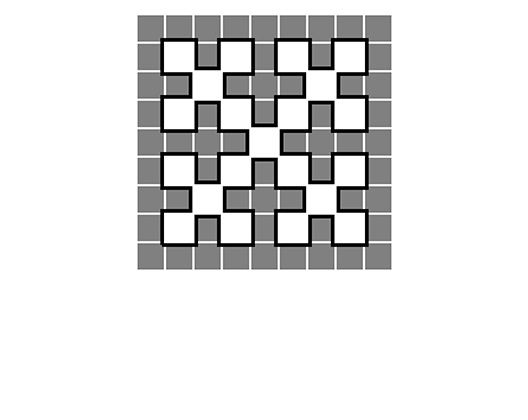Kahle and Roldán studied a question in extremal topological combinatorics: what is the maximal number of holes that a polyomino with n tiles can enclose? They proved that a certain infinite sequence of polyominoes is optimal. The interior boundary of the sequence converges to a fractal space-filling curve. Image by Matthew Kahle and Érika Roldán.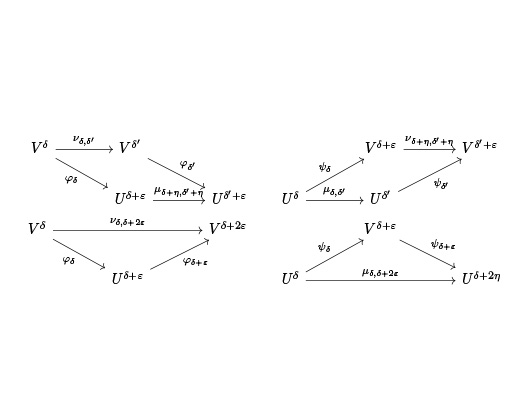Interleavings of persistent modules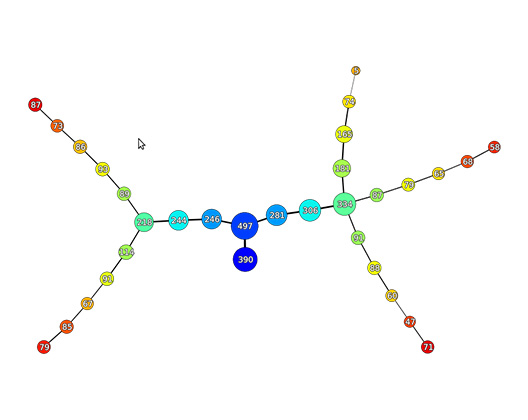A typical graph produced by the Mapper algorithm.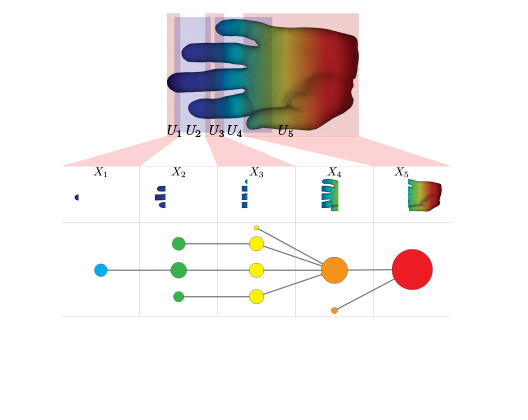A pictorial explanation of the mapper algorithm. Image by G.Carlsson, G. Singh, and F. Memoli.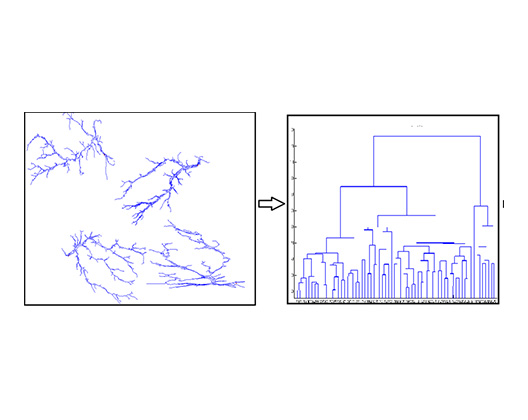A summary of the neuronal connectivity paths extracted from the AAV tracer (3D image) data as computed by a discrete Morse theory based framework, developed by S. Wang, Y. Wang and P. Mitra.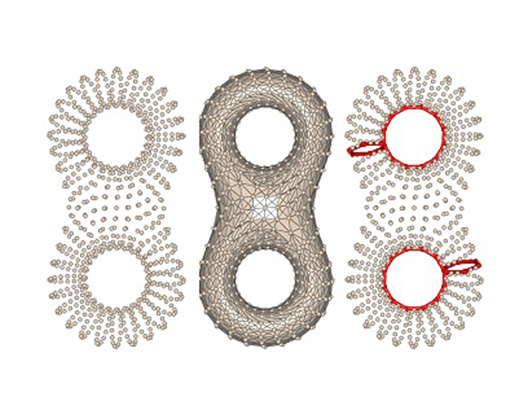Shortest loops for one dimensional homology from point cloud data by the algorithm of Dey, Sun, Wang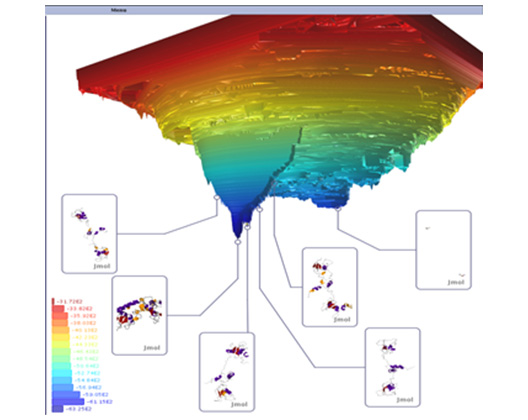A 2D topological landscape metaphor for the high dimensional protein energy landscape, computed via the topological concept of contour trees, developed by W. Harvey, Y. Wang and collaborators. This provides a visual analytic platform for exploration of the large collection of molecular simulation data (for molecule surviving in the picture).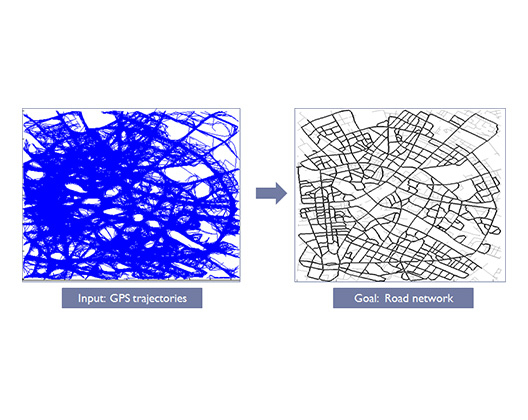Road network automatically reconstructed from a collection of more than 17K noisy GPS trajectories, based on a persistence-guided discrete Morse theory based framework, developed by S. Wang, Y. Wang and Y. Li.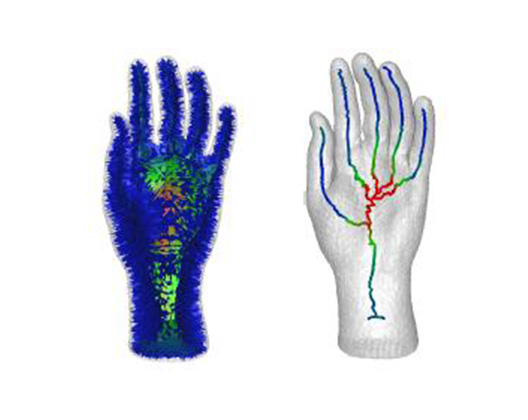Curve skeletons by medial geodesic function based on the work of Dey and Sun.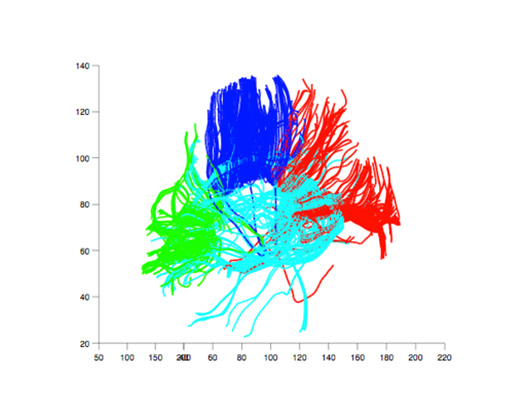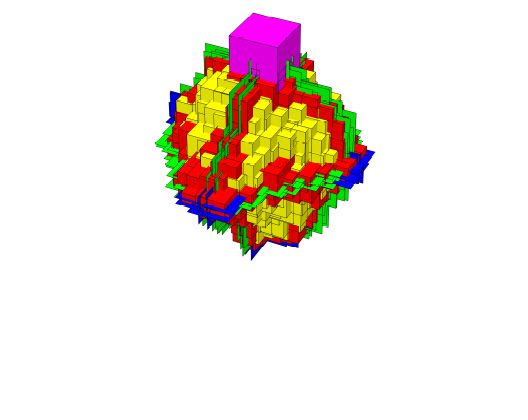Gravner, Holroyd and Sivakoff study threshold-growth dynamics on the 3-dimensional cubic lattice with "obstacles" --- removed vertices. When the obstacles are too numerous, they prevent the threshold-3 growth process from reaching the origin. Depicted here is the blocking structure formed by the randomly placed obstacles, which is impenetrable by the growth process. Image by Alexander Holroyd.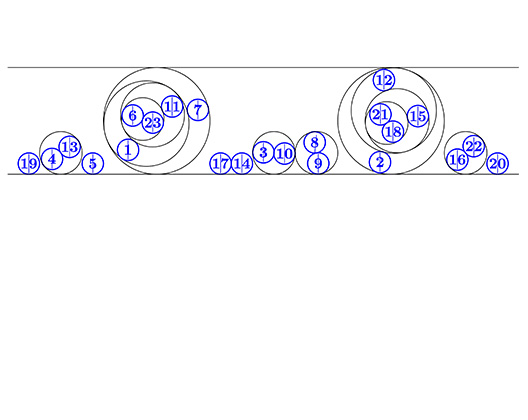Kahle and MacPherson studied the configuration space of disks in an infinite strip in the plane. The image represents one point on a 12-dimensional cycle in the 46-dimensional configuration space config(23,5).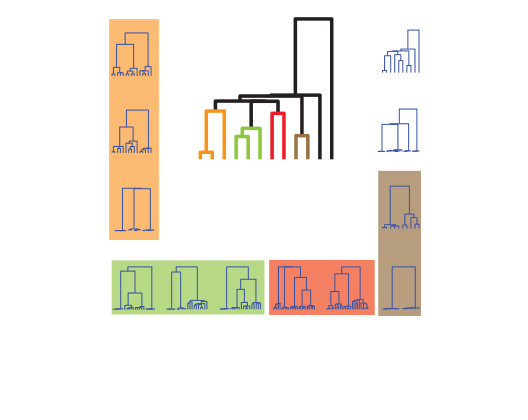Hierarchical clustering on the space of dendrograms. Image by F. Memoli.

Our  interests span the following topics:

• clustering algorithms and Reeb graph / dendrogram reconstruction
• computational topology
• surface/manifold reconstruction and mesh generation
• computing optimal homology generators
• metric measure spaces and shape matching
• low-distortion metric embeddings
• Morse theory
• random graphs and percolation theory
• random topology
• shape analysis
• topological graph theory
• geometric approximation algorithms
• persistent homology and cohomology, and applications
• spectral and geometric methods in machine learning and data analysis
• geometric and topological methods in data analysis